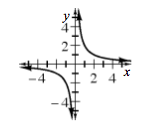### Home > A2C > Chapter 1 > Lesson 1.2.2 > Problem1-93

1-93.

Create multiple representations of the function $g ( x ) = \frac { 2 } { x }$. Then make as many summary statements as you can.

What happens to the value of $y$, when $x = 0$? What is the domain?

Is there any number that cannot be an output? What is the range?

Does this graph have $x$- or $y$-intercepts? Why?

What can you say about symmetry?

Make sure to justify your summary statements using multiple representations.

Use the eTool below to create multiple representations of the function.
Click the link at right for the full version of the eTool: A2C 1-93 HW eTool

 $x$ $y$ $-3$ $-\frac{2}{3}$ $-2$ $-1$ $-1$ $-2$ $-0.5$ $-4$ $0$ undef. $0.5$ $4$ $1$ $2$ $2$ $1$ $3$ $\frac{2}{3}$